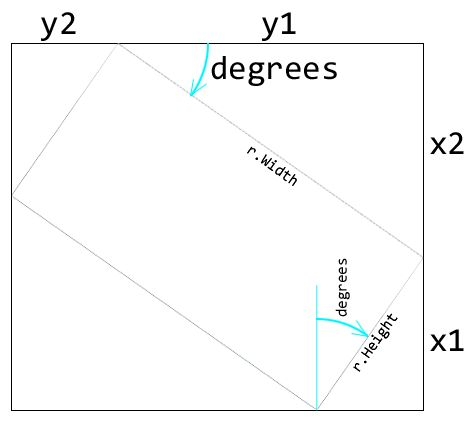## Sunday, January 23, 2011

### How to Determine the Bounding Rectangle of a Rotated Element

I am currently working on a Silverlight application where one of the UI elements is “pinned” to the edges in such a way that when the user resizes their browser, the element will scale to fill the space. The trick to its scale, however is that I want the proportions to remain the same.

In my proof of concept, this element was a simple 4:3 rectangle. In order to determine how to resize it, I would calculate the percentage of scaling needed to fill the space based on the height of my rectangle and the scaling need to fill the space based on the width. I would use the smaller of the two percentages to ensure that the resized rectangle would always fit in both height and width.

When I went to implement the actual application, I had reworked the UI so that the rectangle (which represents a photo snapshot) was rotated by 15 degrees to give it a scrapbook type look.

For most scenarios, my math held relatively well, however in some resizing, the lower left corner or upper right corners would scale off the canvas. At first I didn’t realize what had changed, but then it occurred to me that the “true” heights and widths of my scaled rectangle would technically fit on the canvas, but my rectangle was now rotated, which sometimes caused the corners to rotate off the screen.

Today I sat down and created some methods that would determine the bounding box of my rotated rectangle. Now I can use the same math but with the bounding rectangle instead of the rotated rectangle. This ensures my photo rectangle will always fit on screen.

To determine the bounding rectangle we will need to make 4 calculations using basic algebra. Two for height and two for width. Consider the following diagram:If you remember your algebra (I didn’t, I had to look it up here) you remember that Sin = Opposite/Hypotenuse. This is pretty much all we need if we consider the x1, x2, y1, and y2 values we need to be “opposites” and the height and width of our rectangle to be the hypotenuse.

So for x1 we use the formula SIN(90-degrees) * r.Height. We use 90 – degrees because the triangle with r.Height as our hypotenuse and x1 as our opposite has an angle of 90 minus the degree of rotation of our rectangle.

For x2 we can use SIN(degrees) * r.Width.

For y1 we use SIN(90-degrees) * r.Width.

And finally for y2, SIN(degrees) * r.Height.

That seems simple enough. But in C# there is a small wrinkle. The Math.Sin() method takes radians as a parameter instead of the degrees we use for the rotation  transform. In order to convert our degrees to radians, we multiply by a coefficient of 0.0174532925.

I also added some logic so that I can simply pass my methods an abstract FrameworkElement and determine if the element has a rotation transform applied. If not the plain height and width of the element will do.

So given two XAML rectangles, rectangle1 and rectangle2, where rectangle1 is my source rectangle and rectangle2 represents the bounding rectangle, my code to calculate the bounding rectangle looks something like this:

`   1:  namespace SilverlightApplication1`
`   2:  {`
`   3:      public partial class MainPage`
`   4:      {`
`   5:          public MainPage()`
`   6:          {`
`   7:              InitializeComponent();`
`   8:   `
`   9:              rectangle2.Height = GetBoundingHeight(rectangle1);`
`  10:              rectangle2.Width = GetBoundingWidth(rectangle1);`
`  11:          }`
`  12:   `
`  13:          private static double GetBoundingHeight(FrameworkElement rectangle)`
`  14:          {`
`  15:              if(HasRotationTransform(rectangle))`
`  16:              {`
`  17:                  return rectangle.Height;`
`  18:              }`
`  19:              var degrees = ((CompositeTransform) rectangle.RenderTransform).Rotation;`
`  20:              return Math.Sin(ToRadians(90 - degrees)) * rectangle.Height + Math.Sin(ToRadians(degrees)) * rectangle.Width;`
`  21:          }`
`  22:   `
`  23:          private static double GetBoundingWidth(FrameworkElement rectangle)`
`  24:          {`
`  25:              if (HasRotationTransform(rectangle))`
`  26:              {`
`  27:                  return rectangle.Width;`
`  28:              }`
`  29:              var degrees = ((CompositeTransform) rectangle.RenderTransform).Rotation;`
`  30:              return Math.Sin(ToRadians(90 - degrees)) * rectangle.Width + Math.Sin(ToRadians(degrees)) * rectangle.Height;`
`  31:          }`
`  32:   `
`  33:          private static bool HasRotationTransform(FrameworkElement rectangle)`
`  34:          {`
`  35:              return rectangle.RenderTransform == null || rectangle.RenderTransform.GetType() != typeof(CompositeTransform);`
`  36:          }`
`  37:   `
`  38:          private static double ToRadians(double degrees)`
`  39:          {`
`  40:              const double radianConversionConstant = 0.0174532925;`
`  41:              return degrees*radianConversionConstant;`
`  42:          }`
`  43:      }`
`  44:  }`

Enjoy!

#### 1 comment:

1.It really disappointed me that VisualTreeHelper.GetContentBounds or VisualTreeHelper.GetDescendantBounds is not included in Silverlight but are there for WPF.